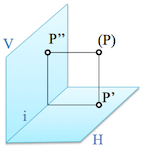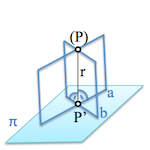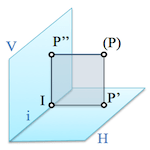# Fundamentals del System dihedralWe have seen the present Representation Systems descriptive geometry that is the set of techniques that allows to represent geometric character three dimensional space on a two dimensional surface.

In particular we explore in detail the so-called “System dihedral” (view classification systems of representation) based on the prospects relationships appearing in the cylindrical projection on two planes orthogonal projection.

## Orthogonal projection

To understand in depth based on orthogonal cylindrical projection model previously review some theorems to facilitate spatial analysis we.

A line is perpendicular to a plane if it is with two parallel lines contained in the plane.The projection of a point (P) space on a given projection plane is obtained by determining the line “r” containing the point and perpendicular to this plane, thus any two lines perpendicular to “to” and “b” that are not parallel to said plane.

The projection P’ will be the intersection of the line r with the plane.

Another space theorem which will be useful is the following:

If a line is perpendicular to a plane, all planes that contain it are also orthogonal to the plane.If the line r perpendicular to the plane, the planes determined by the lines through P’ (to, b, etc.) planes are orthogonal to the first.

We may think that the straight line r is the hinge of a door and flat infinite positions occupied by turning on its axis.

Finally we need to establish a relationship between three orthogonal planes each:

If a plane is perpendicular to two other planes, it is at the intersection of these straight.The straight intersection of two planes, i, is the common address to both planes. The three planes intersect at a point I.

If we project orthogonally the point (P) on planes H and V.., straight (P)-P’ and (P)-P” They are orthogonal to them respectively.

The plane containing a (P)-P’ Sera ortogonal to plan H and similarly, the plane containing the straight (P)-P” it will be a V... Therefore, if we consider the plane formed by the points (P)P’P” and point I, this will be perpendicular to the V.. and H and hence their common direction, straight i.

This latter property allows us to establish the perspective relationship between two projections linked.

## System dihedral

If we folded down the horizontal plane of projection on the vertical plane ( o al revés), we can see the two projections on the same plane.By rotating the horizontal plane H on the vertical V.. obtain two orthogonal projections on the same plane that will match the drawing.

This model representation is known from “System dihedral” As we will need two orthogonal projections on planes, at least, to unambiguously determine the spatial positions of the points represented.

The process of “restitution” Space should be allowed to know how they are located in space geometric elements represented in this system.

We can see that when folding the horizontal plane about the vertical, projections P’ and P” are aligned on a line perpendicular to the intersection i both planes. This line is called “Baseline” between the projections of the points. The straight i is known by the name of “Ground line

Reference lines between two views are orthogonal to the corresponding ground line.

We'll see what we can do without the land line when we develop the system. For now it serves to understand the essence of the.We can label differently from projections on planes. Some biographies use subscripts, other accents or Roman numerals.

Normally the projection on the horizontal plane is known as “first” projection, Guests are on the vertical “second” and a third plane orthogonal to the above, called profile plane, we “third” projection.

Next Lesson … Projection of the line